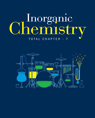Click to Chat

1800-1023-196

+91-120-4616500

CART 0

• 0

MY CART (5)

Use Coupon: CART20 and get 20% off on all online Study Material

ITEM
DETAILS
MRP
DISCOUNT
FINAL PRICE
Total Price: Rs.

There are no items in this cart.
Continue Shopping
`        kindly tell que 19...... density of o2......fast plxxx`
5 months ago

```							the density of molecules of gas : ρ = P * M/R * T a) at STP ( P = 1.01 * 10^5 Pa T = 273 K) M O2 = 32 g/mole = 0.032 kg/mole ρ = P * M/R * T = (1.01 * 10^5 * 0.032)/(8.31 * 273 ) = 1.425 kg/m^3 b) at P = 2 atm = 273 K ρ = P * M/R * T = (2 * 1.01 * 10^5 * 0.032)/(8.31 * 273 ) =2.849 kg/m^3 c) ρ = P * M/R * T = (4 * 1.01 * 10^5 * 0.032)/(8.31 * 546 ) = 2.848 kg/m^3 d) ρ = P * M/R * T = (3 * 1.01 * 10^5 * 0.032)/(8.31 * 546 ) =2.136 kg/m^3 Hence option A is correct
```
5 months ago
Think You Can Provide A Better Answer ?

## Other Related Questions on Inorganic Chemistry

View all Questions »### Course Features

• 731 Video Lectures
• Revision Notes
• Previous Year Papers
• Mind Map
• Study Planner
• NCERT Solutions
• Discussion Forum
• Test paper with Video Solution### Course Features

• 54 Video Lectures
• Revision Notes
• Test paper with Video Solution
• Mind Map
• Study Planner
• NCERT Solutions
• Discussion Forum
• Previous Year Exam Questions